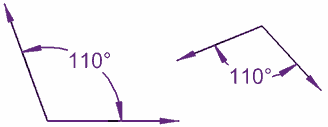+

# Angles

##### Rating:
(7)
• (7)
• (0)
• (0)
• (0)
• (0)
Author: Kyle Webb
##### Description:
• Review congruent segments
• Define a "ray"
• Define an "angle"
• Classifying types of angles
• Define "congruent angles"
• Define "angle bisectors"
• Look at angle relationships when two lines intersect

In the first unit we developed an understanding of congruence as it relates to line segments.  We introduced the symbol for congruence and discussed measurement in respect to finding the length of a line segment.  In this section we will further develop our understanding of congruence and measurement as we begin to work with angles.  We will also use what we have learned about deductive reasoning and the introduction to the postulates to make specific statements about angles.  Much of what is included in this section are definitions.

(more)
Tutorial

## Review of Congruent Segments

Take a look back at what congruent segments are.

What is a ray?

## Angles

What is an angle?

## Congruent Angles

Just like line segments, angles can be congruent.

Two angles are congruent when they have the same measure.

Example:We can show that two angles are congruent by using a little "tick" like we do with congruent line segments.

To see an example, look at anglesand angles## Angle Bisectors

If a ray divides a larger angle into two smaller, congruent angles, it is called an angle bisector.## Angle Relationships

When two lines intersect, they form some angles. These are some of the relationships between those angles.

## Complementary & Supplementary Angles

Two angles that sum up toare called complementary angles.(Angles x and y are complementary angles)

Two angles that sum up toare called supplementary angles.(Angles a and b are supplementary angles)

Rating Header